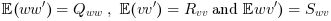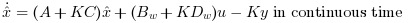Scilab Home page | Wiki | Bug tracker | Forge | Mailing list archives | ATOMS | File exchange
Change language to: English - Português - 日本語 - Русский

# lqe

### Syntax

[K, X] = lqe(Pw)
[K, X] = lqe(P, Qww, Rvv)
[K, X] = lqe(P, Qww, Rvv, Swv)

### Arguments

Pw

A state space representation of a linear dynamical system (see syslin)

P

A state space representation of a linear dynamical system (nu inputs, ny outputs, nx states) (see syslin)

Qww

Real nx by nx symmetric matrix, the process noise variance

Rvv

full rank real ny by ny symmetric matrix, the measurement noise variance.

Swv

real nx by ny matrix, the process noise vs measurement noise covariance. The default value is zeros(nx,ny).

K

a real matrix, the optimal gain.

X

a real symmetric matrix, the stabilizing solution of the Riccati equation.

### Description

This function computes the linear optimal LQ estimator gain of the state estimator for a detectable (see dt_ility) linear dynamical system and the variance matrices for the process and the measurement noises.

#### Syntax [K,X]=lqe(P,Qww,Rvv [,Swv])

Computes the linear optimal LQ estimator gain K for the dynamical system:orWhere w and v are white noises such asThe values of B and D are not taken into account.##### Standard formThis covariance matrix can be factored using full-rank factorization (see fuffrf) asAnd consequently the initial dynamical system is equivalent toorWhere w is now a white noise such as#### Syntax [K,X]=lqe(Pw)

Computes the linear optimal LQ estimator gain K for the dynamical systemWhere w is a white noise with unit covariance.

#### Properties

• A + K.C is stable.

• the state estimator is given by the dynamical system:orIt minimizes the covariance of the steady state error.

• For discrete time systems the state estimator is such that:(one-step predicted x).

#### Algorithm

Let Q = BwBw', R = DwDw' and S = BwDw'

• For the continuous time case K is given by: K = -(X.C'+ S).R-1

where X is the solution of the stabilizing Riccati equation

(A - SR-1C)X + X(A - SR-1C)' - XC'R-1CX + Q - SR-1S'= 0

• For the discrete time case K is given by K = - (A X C'+S)(C X C'+ R)+

where X is the solution of the stabilizing Riccati equation

AXA'- X - (AXC' + S)(CXC' + R)+(CXA' + S') + Q

### Examples

Assume the dynamical system formed by two masses connected by a spring and a damper:A force(where e is a noise) is applied to the big one, the deviations from equilibrium positions dy1 and dy2 of the masses are measured. These measures are also subject to an additional noise v.

A continuous time state space representation of this system is:and e and v are discrete time white noises such asThe instructions below can be used to compute a LQ state observer of the discretized version of this dynamical system.

// Form the state space model
M = 1; m = 0.2; k = 0.1; b = 0.004;
A = [  0     1    0    0
-k/M  -b/M  k/M  b/M
0     0    0    1
k/m  b/m  -k/m  -b/m];
B = [0; 1/M; 0; 0];
C = [1 0 0 0  //dy1
0 0 1 0];//dy2
//inputs u and e; outputs dy1 and dy2
P = syslin("c",A, B, C);
// Discretize it
dt=0.5;
Pd=dscr(P, dt);

//  Set the noise covariance matrices
R_vv=0.001*eye(2,2); //measurement noise variance
Q_ww=Pd.B*Q_e*Pd.B'; //input noise adds to regular input u

//----syntax [K,X]=lqe(P,Qww,Rvv [,Swv])---
Ko=lqe(Pd,Q_ww,R_vv); //observer gain

//----syntax [K,X]=lqe(P21)---
bigR =blockdiag(Q_ww, R_vv);
[W,Wt]=fullrf(bigR);
Bw=W(1:size(Pd.B,1),:);
Dw=W(($+1-size(Pd.C,1)):$,:);
Pw=syslin(Pd.dt,Pd.A,Bw,Pd.C,Dw);
Ko1=lqe(Pw); //same observer gain

//Check gains equality
norm(Ko-Ko1,1)

// Form the observer
O=observer(Pd,Ko);
//check stability
and(abs(spec(O.A))<1)
// Check by simulation
// Modify Pd to make it return the state
Pdx=Pd;Pdx.C=eye(4,4);Pdx.D=zeros(4,1);
t=0:dt:30;
u=zeros(t);
x=flts(u,Pdx,[1;0;0;0]);//state evolution
y=Pd.C*x;
// simulate the observer
x_hat=flts([u+0.01*rand(u);y+0.0001*rand(y)],O);
clf;
subplot(2,2,1)
plot2d(t',[x(1,:);x_hat(1,:)]'),
e=gce();e.children.polyline_style=2;
L=legend('$x_1=dy_1$', '$\hat{x_1}$');L.font_size=3;
xlabel('Time (s)')
subplot(2,2,2)
plot2d(t',[x(2,:);x_hat(2,:)]')
e=gce();e.children.polyline_style=2;
L=legend('$x_2=dy_1^+$', '$\hat{x_2}$');L.font_size=3;
xlabel('Time (s)')
subplot(2,2,3)
plot2d(t',[x(3,:);x_hat(3,:)]')
e=gce();e.children.polyline_style=2;
L=legend('$x_3=dy_2$', '$\hat{x_3}$');L.font_size=3;
xlabel('Time (s)')
subplot(2,2,4)
plot2d(t',[x(4,:);x_hat(4,:)]')
e=gce();e.children.polyline_style=2;
L=legend('$x_4=dy_2^+$', '$\hat{x_4}$');L.font_size=3;
xlabel('Time (s)')### Reference

Engineering and Scientific Computing with Scilab, Claude Gomez and al.,Springer Science+Business Media, LLC,1999, ISNB:978-1-4612-7204-5

• lqr — LQ compensator (full state)
• observer — observer design
• obscont — observer based controller
• lqg — LQG compensator
• fullrf — factorisation de rang plein

### History

 Version Description 6.0 lqe(P,Qww,Rvv) and lqe(P,Qww,Rvv,Swv) syntaxes added.